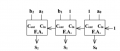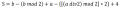#### NotAlive

Joined Dec 28, 2019
1Hello, I would like to get help in this question :

a=a2a1a0 , b=b2b1b0, a>b
why s equals to above?

Thank you#### Papabravo

Joined Feb 24, 2006
18,846
View attachment 195462View attachment 195463
Hello, I would like to get help in this question :

a=a2a1a0 , b=b2b1b0, a>b
why s equals to above?

Thank youHow do you know that it does?
What approaches are available to you?
What approach would you like to take in verifying the statement, or alternatively proving it false?

#### WBahn

Joined Mar 31, 2012
26,526

Start with being sure how to combine and dissect the values and their bits.

If a = a2a1a0, then mathematically

a = (2^2)·a2 + (2^1)·a1 + (2^0)·a0
a = 4·a2 + 2·a1 + 1·a0

and similar for the other two.

Now, given 'a', how could you pick off each of its bits using div (integer division) and mod (remainder after integer division) operations?

Once you have that, then you can either piece together the equation they have, or simply brute force the result from the circuit.

Perhaps it might help to group and slightly rewrite the result as

S = [b - (b mod2)] + [a - {[(a div 2) mod 2[ * 2}] + [3 + 1]

#### Papabravo

Joined Feb 24, 2006
18,846